# 基于截枝随机森林模型的TMS特征选择方法研究TMS Feature Selection Method for Truncate Based Random Forest Model

DOI: 10.12677/CSA.2020.102029, PDF, HTML, XML, 下载: 278  浏览: 482

Abstract: TMS has some problems such as inconsistent accounts, wrong data input, missing data, and so on. It needs to analyze and re-classify a lot of data, and to improve the accuracy of classification learning, it needs to select a lot of data features effectively. In this paper, the stochastic forest model is applied to feature selection, according to the number of decision trees, the criteria of feature partition, the maximum feature number in the candidate subset of feature partition, the change of the accuracy of the model after feature rearrangement, etc. , an optimized random forest feature selection method for TMS data is proposed and verified by experiments.

1. 引言

2. 随机森林模型

2.1. 特征划分选择

1) 基于信息增益度量的特征划分选择 信息增益的计算过程：

$Ent\left(D\right)=-\underset{k=1}{\overset{|Y|}{\sum }}{p}_{k}{\mathrm{log}}_{2}{p}_{k}$ (1)

$Gain\left(D,a\right)=Ent\left(D\right)-{\sum }_{v=1}^{V}\frac{|{D}^{v}|}{|D|}Ent\left({D}^{v}\right)$ (2)

2) 基于基尼指数度量的特征划分选择

$Gini\left(D\right)={\sum }_{k=1}^{|Y|}{\sum }_{{k}^{\prime }\ne k}{p}_{k}{p}_{{k}^{\prime }}=1-{\sum }_{k=1}^{|Y|}{p}_{k}^{2}$ (3)

$Gini\text{_}index\left(D,a\right)={\sum }_{v=1}^{V}\frac{|{D}^{v}|}{|D|}Gini\left({D}^{v}\right)$ (4)

${a}_{*}=\mathrm{arg}{\mathrm{min}}_{a\in A}Gini\text{_}index\left(D,a\right)$ (5)

2.2. Bagging算法

Bagging算法是基于自助采样法(boostrap sampling)的一种集成学习方法。给定一个包含n个样本数据的样本集，从中随机取出一个样本放入采样集，之后再把该样本放入原样本集，这样便使得该样本在下一次采样过程中仍有被选中的可能性。重复n次上述采样过程，便获得一个包含n个样本的采样集，且原数据集中的部分样本可能多次出现在采样集中，部分样本可能从未出现。样本在n次采样过程中始终不被采到的概率为 ${\left(1-1/n\right)}^{n}$，取极限可以得到：

$\underset{n\to \infty }{\mathrm{lim}}{\left(1-\frac{1}{n}\right)}^{n}\to \frac{1}{\text{e}}\approx 0.368$ (6)

2.3. 随机森林

${H}^{oob}\left(x\right)=\underset{y\in Y}{\mathrm{arg}\mathrm{max}}\underset{t=1}{\overset{T}{\sum }}I\left({h}_{t}\left(x\right)=y\right)\cdot I\left(x\notin {D}_{t}\right)$ (7)

${ϵ}^{oob}=\frac{1}{|D|}{\sum }_{\left(x,y\right)\in D}I\left({H}^{oob}\left(x\right)\ne y\right)$ (8)

① 确定原数据样本集中的样本个数N。

② 确定原数据样本集中的样本特征数目M。

③ 从原数据样本集中随机采样得到T个训练样本子集。

④ 确定决策树的节点属性划分时需要使用的特征个数m，且 $m

⑤ 使用T个训练样本子集，根据确定的划分使用特征个数m，用信息增益、基尼指数等特征重要性度量方法，生成T棵决策树。

⑥ 将T棵决策树进行集成，对预测结果采用简单投票法来得出最终分类结果。

3. 随机森林模型在TMS特征选择中的应用

TMS系统数据具有较高维度，且存在噪声，在进行分类任务之前，必须进行特征选择，否则分类器的精度以及学习效率会异常低下。目前针对TMS系统数据尚需通过人工对照标记来选择学习任务的特征，具有强烈的主观性，缺乏科学的理解与支撑。在此背景下，针对TMS系统数据的特点，用随机森林模型进行特征选择，并分析基于决策树数目、基于特征划分标准、基于特征划分候选子集中的最大特征数等多个参数对模型结果的影响，提出优化的TMS系统数据的随机森林特征选择方法。

TMS系统数据可大致分为实时告警类、维护管理类、资源管理类、设备画像类及外部数据源五类 。由于数据规模的膨胀，TMS系统中的数据具有不同省份分散存储、多个字段缺乏相应解释、数据噪声较多、空值较多等特点。基于上述数据特点，需要对TMS系统的数据进行收集与预处理，并选择适合的模型与方法进行训练，最后进行可视化展现。对于大数据的数据分析关键技术，需要基于其数据类型、数据规模的特点，可以从三个方面着手：

① 从大数据的清洗、整理、抽样以及特征选择的角度入手，将大数据小数据化。

② 开展大数据下各种分类聚类算法的研究，根据具体问题选取特定的机器学习算法。

③ 开展大数据的并行算法，将传统的数据挖掘、数据分析算法用分布式并行实现，提高效率。

3.1. 基于决策树数目

① 在决策树数目较少的范畴，进行迭代测试，观察决策树数目与随机森林模型准确率的关系。

② 在决策树数目达到一定高度后，进行以一定步长的迭代测试，观察决策树数目与随机森林模型准确率的关系。

③ 衡量决策树数目与随机森林模型准确率的关系，择优选择在满足一定准确率的条件下，决策树数目最为合适的大小。

3.2. 基于特征划分标准

① 使用信息增益的特征划分准则，进行随机森林的建模，得到特征重要性程度的排名，以及该模型的准确率。

② 使用基尼指数的特征划分准则，进行随机森林的建模，得到特征重要性程度的排名，以及该模型的准确率 。

③ 对比信息增益与基尼指数所进行特征划分对TMS系统随机森林模型的准确率影响，选取较之更适合于TMS系统的特征划分方法。

3.3. 基于特征划分候选子集中的最大特征数

① 在随机森林模型构建时，选取的特征划分候选子集中的最大特征数，从1到n进行迭代测试，其中n为数据样本集的所有特征数目。

② 对比在特征划分候选子集中的最大特征数不同的情况下，随机森林模型的准确率以及该模型下的特征重要性排名，从而选取最适合于TMS系统的特征划分候选子集中最大特征数。

3.4. 基于特征重排后模型的准确率变化

OOB样本对评估模型准确率有着重要的作用，因此可以通过将OOB样本中的每一个特征的所有特征值进行重排，来影响重排后的随机森林模型准确率。通过衡量重排前后模型准确率的变化幅度，就可以获得该特征对于随机森林模型的重要程度。如果是相对重要的特征，则该特征的特征值顺序变动后，会大幅度降低原模型的准确率；如果是相对不重要的特征，则该特征的特征值顺序变动后，对原模型的准确率不会产生较大的影响。

① 使用原始数据样本集训练得到随机森林模型，记录其模型准确率。

② 使用OOB样本对每一个特征进行所有特征值重排，通过对比重排前后随机森林模型准确率的变化大小，得到新的特征重要性排名。

① 使用基于决策树数目、基于特征划分标准、基于特征划分候选子集中的最大特征数、基于特征重排后模型的准确率变化的参数，得到一个随机森林模型和一组特征重要性排名。

② 对得到的特征重要性排名进行截枝，得到最为重要的前几个特征。

③ 使用新的特征重新训练随机森林模型，并得到一个新的随机森林模型和一组新的特征重要性排名。

④ 可以使用新的随机森林模型对后续的分类任务进行分类。

4. 实验与分析

4.1. 实验数据

Table 1. Summary of business types

4.2. TMS系统数据预处理

4.3. 实验分析

4.3.1. 决策树数目对比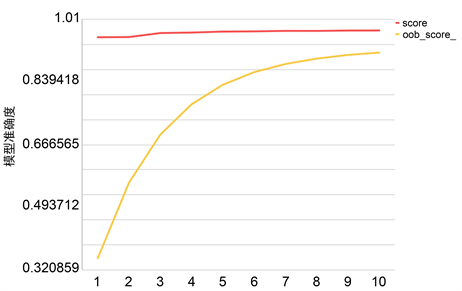Figure 1. The influence of decision tree number on score and oob_score_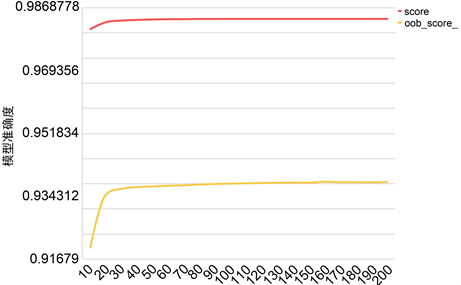Figure 2. Effect of decision tree number on accuracy of random forest model in large data sets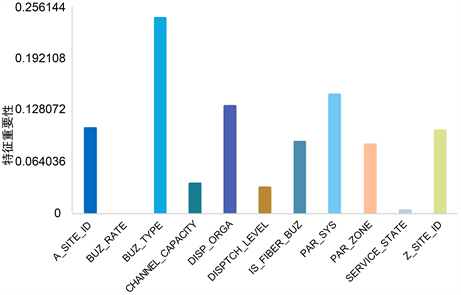Figure 3. The importance degree of features when the number of decision trees is 70

4.3.2. 特征划分标准对比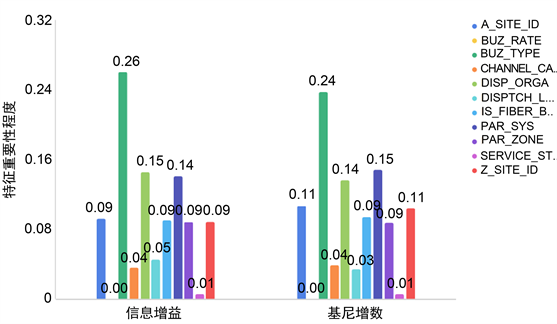Figure 4. The importance of characteristics of different criteria

4.3.3. 特征划分候选子集中的最大特征数对比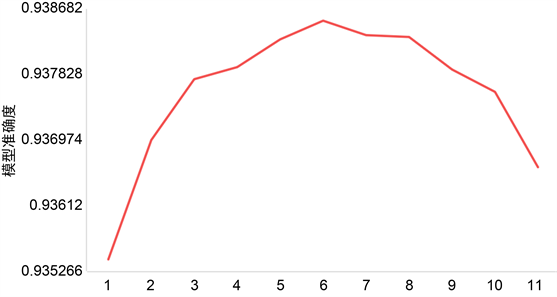Figure 5. Effect of maximum characteristic number on model accuracy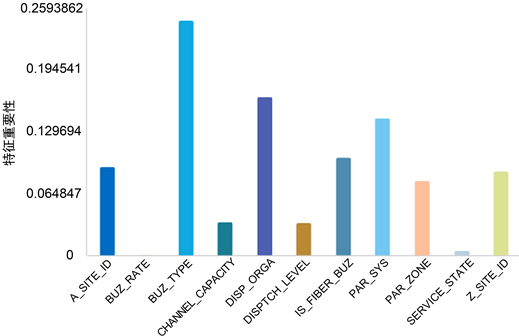Figure 6. The degree of feature importance when the maximum feature number is 6

4.3.4. 特征重排的留出法验证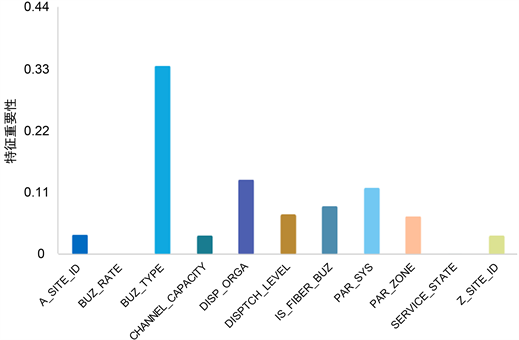Figure 7. Importance degree of feature based on feature rearrangement

4.3.5. 特征截枝前后对比Table 2. Three-dimensional characteristic truncation results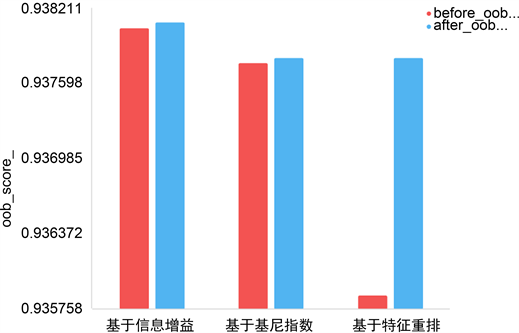Figure 8. Comparison of oob models before and after feature truncation in three dimensions

① 随机森林模型的决策树数目取值为70。

② 对于随机森林模型中的每一棵基决策树，使用信息增益的方法来对节点进行特征划分。

③ 在基决策树的每一个节点进行特征划分时，随机选取的特征划分子集中的最大特征数为6。

④ 在对特征的重要性程度进行排名时，采用特征重排后随机森林模型准确率变化程度作为衡量标准。

⑤ 对得到的特征重要性排名进行截枝，去除特征重要性程度小于0.1的特征，并用剩余的特征重新进行①至④的过程，最终得到优化过的特征重要性排名和一个可以直接用于分类任务的随机森林模型。Table 3. Importance ranking of features

5. 结语

  Quinlan, J.R. (1986) Induction of Decision Trees. Kluwer Academic Publishers, New York, 22-26. https://doi.org/10.1007/BF00116251  Breiman, L.I., Friedman, J.H., Olshen, R.A., et al. (1984) Classification and Regression Trees (CART). Encyclopedia of Ecology, 40, 582-588. https://doi.org/10.2307/2530946  Surhone, L.M., Tennoe, M.T., Henssonow, S.F., et al. (2010) ID3 Algo-rithm. Betascript Publishing, New York, 132-133.  Steven, L. (1994) Book Review: C4.5: Programs for Machine Learning by J. Ross Quinlan. San Francisco, USA: Morgan Kauffman Publishers Inc., 1993. Machine Learning, 16, 87-92. https://doi.org/10.1007/BF00993309  Jiang, W. (2004) Process Consistency for Adaboost. Annals of Statistics, 32, 13-29. https://doi.org/10.1214/aos/1079120128  Breiman, L. (1996) Bagging Predictors. Machine Learning, 24, 123-140. https://doi.org/10.1007/BF00058655  Breiman, L. (2001) Random Forests. Machine Learning, 45, 5-32. https://doi.org/10.1023/A:1010933404324  Efron, B. and Tibshirani, R. (1986) Bootstrap Methods for Standard Errors, Confidence Intervals, and Other Measures of Statistical Accuracy. Statistical Science, 1, 54-75. https://doi.org/10.1214/ss/1177013815  胡志鹏, 颜秉勇, 彭亦功. 层次采样的代价敏感随机森林算法及其应用[J]. 计算机工程与设计, 2019, 40(12): 3361-3366.  李春生, 焦海涛, 刘澎, 等. 基于C4.5决策树分类算法的改进与应用[J]. 计算机技术与发展, 2020(4): 1-9.  刘凯, 郑山红, 蒋权, 等. 基于随机森林的自适应特征选择算法[J]. 计算机技术与发展, 2018, 28(9): 101-104.  杨晶, 廖翯, 妥建军. 面向智能电网应用的电力大数据关键技术[J]. 电子技术与软件工程, 2018(4): 173.  文武, 赵成, 赵学华, 等. 基于信息增益和萤火虫算法的文本特征选择[J]. 计算机工程与设计, 2019, 40(12): 3457-3462.  陈谌, 梁雪春. 基于基尼指标和卡方检验的特征选择方法[J]. 计算机工程与设计, 2019, 40(8): 2342-2345.  罗计根, 杜建强, 聂斌, 等. 一种聚类欠采样策略的随机森林优化方法[J]. 计算机工程与应用, 1-9. http://kns.cnki.net/kcms/detail/11.2127.TP.20191125.0924.002.html